# Nerfies Deformable Neural Radiance Fields

Nerfies: Deformable Neural Radiance Fields present the first method capable ofphotorealistically reconstructing deformable scenes using photos/videos cap- tured casually from mobile phones. Our approach augments neural radiance fields (NeRF) by optimizing an additional continuous volumetric deformation field that warps each observed point into a canonical 5D NeRF. To avoid local minima, we propose a coarse-to-fine optimization method for coordinate-based models that allows for more robust optimization. To avoid overfit, we propose an elastic regularization ofthe deformation field that further improves robustness.

Figure below shows an overview of the Nerfies. Piror to vanilla NeRF, Nerfies add a neural network (neural deformation fields) to deform the coordinate in observation frame to cannoical frame, which then could be used by vanilla NeRF.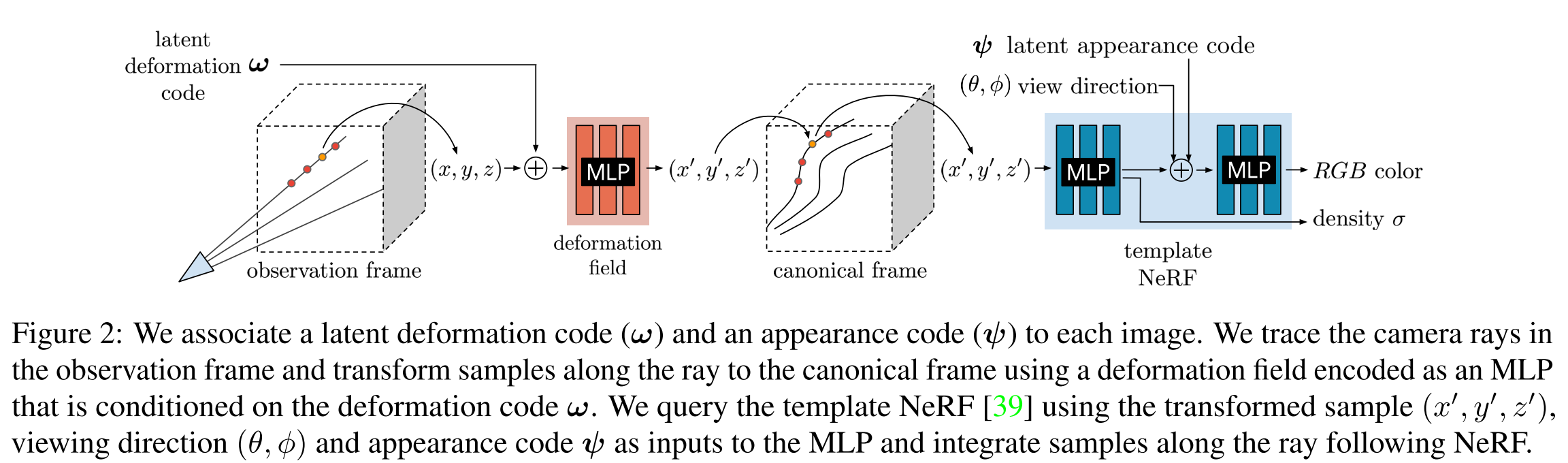Why: NeRF has difficulty in modeling people with hand-held cameras due both to 1) nonrigidity – our inability to stay perfectly still, and 2) challenging materials like hair, glasses, and earrings that violate assumptions used in most recon- struction methods.

# Neural Deformation Fields

Related work: DynamicFu- sion  and Neural Volumes  also model a template and a per-frame deformation, but the deformation is defined on mesh points and on a voxel grid respectively, whereas we model it as a continuous function using an MLP.

We model the deformation fields for all time steps using a mapping $$T : (x, w_i) \to x'$$, which is conditioned on a per-frame learned latent deformation code $$w_i$$. Each latent code encodes the state of the scene in frame i. Thus we have

$G(x,d,\psi_i,w_i)=F(T(x,w_i),d,\psi_i)$

A simple model of deformation is a displacement field $$V : (x, w_i) \to t$$, defining the transformation as $$T(x, w_i) = x +V(x, wi)$$. This formulation is sufficient to represent all continuous deformations; however, rotating a group of points with a translation field requires a different translation for each point, making it difficult to rotate regions of the scene simultaneously. We therefore formulate the deformation using a dense SE(3) field $$W : (x, w_i) \to (r,v)\mbox{ where } r\in SE(3)$$. An SE(3) transform encodes rigid motion, allowing us to rotate a set of distant points with the same parameters.

As mentioned before, we encode the transformation field in an MLP $$W : (x, w_i) \to (r,v)$$ using a NeRF-like architecture, and represent the transformation of every frame i by conditioning on a latent code $$w_i$$. Check the image below for detailed architecture.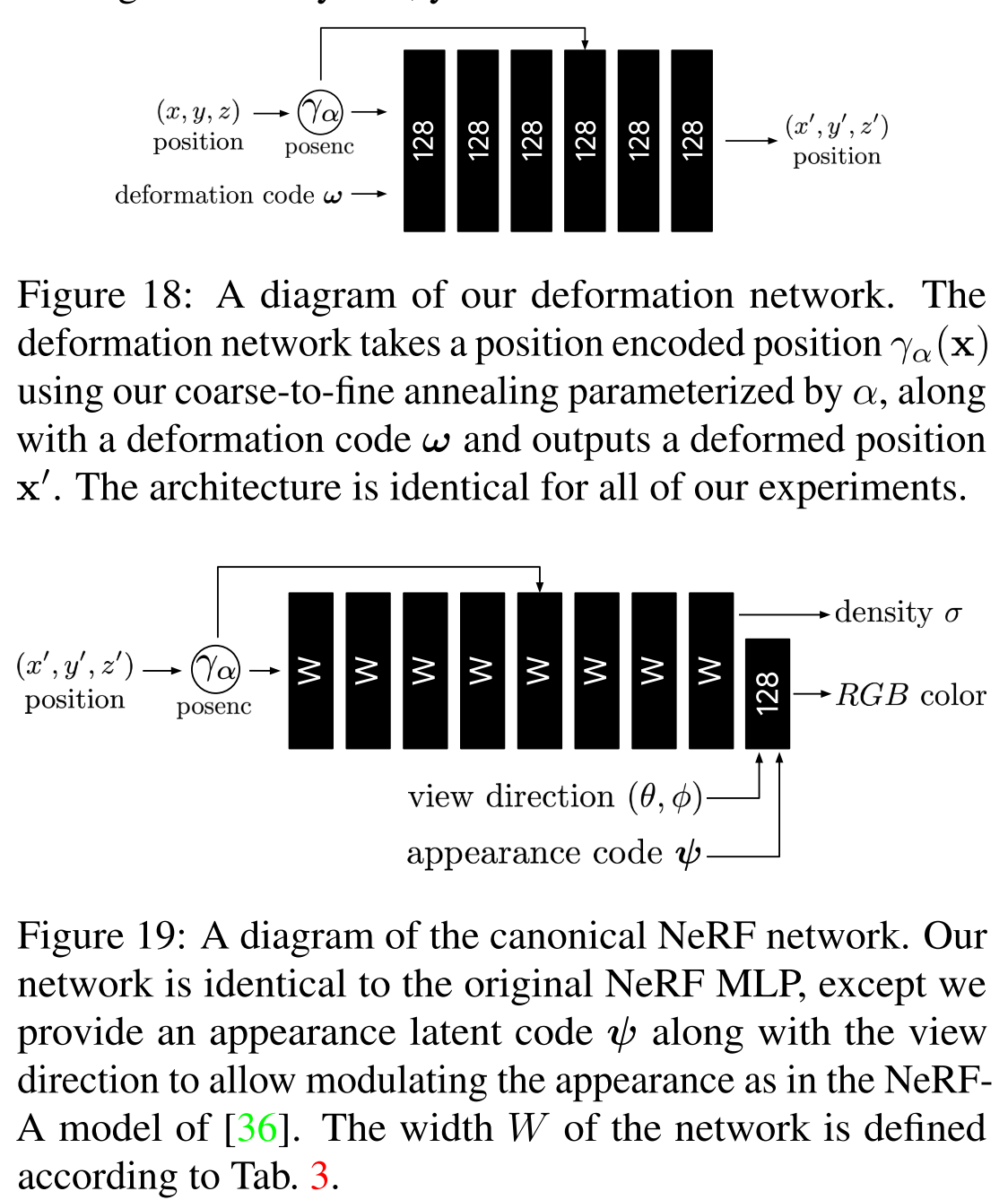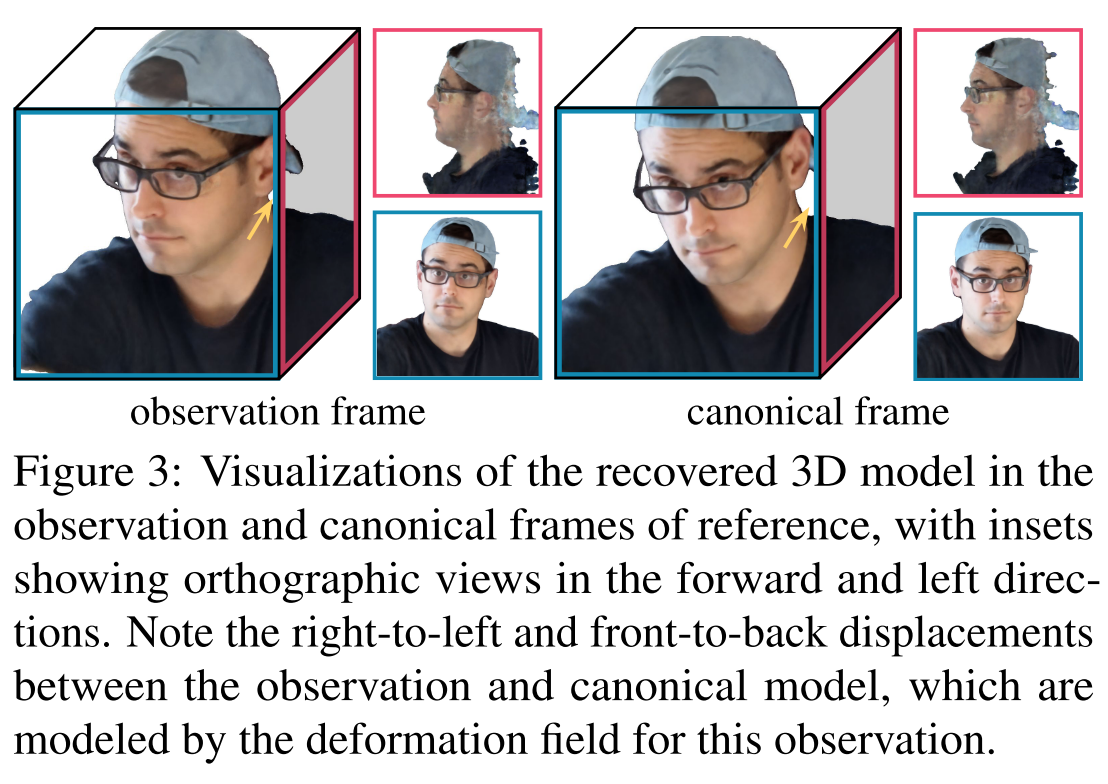# Elastic Regularization

The deformation field adds ambiguities that make optimization more challenging. It is therefore crucial to introduce priors that lead to a more plausible solution. It is common in geometry processing and physics simulation to model non-rigid deformations using elastic energies measuring the deviation of local deformations from a rigid motion.

The Jacobian $$J_T(x)$$ of this mapping at a point $$x \in \mathbb{R}^3$$ describes the best linear approximation of the transformation at that point. We can therefore control the local behavior of the deformation through $$J_T$$. We write the elastic regularization as:

$L_{elastic}(x)=\lVert log\Sigma -log I\rVert_F = \lVert log\Sigma\rVert_F$

Here $$\Sigma$$ is the singular value decomposition of $$J_T=U\Sigma V$$. This regularization pushes for a rigid rotation.

Although humans are mostly rigid, there are some movements which can break our assumption of local rigidity, e.g., facial expressions which locally stretch and compress our skin. We therefore remap the elastic energy defined above using a robust loss:

$L_{elastic}(x)=\rho(\lVert log\Sigma\rVert_F,c)\mbox{ where }\rho(x,c)=\frac{2(\frac{x}{c})^2}{(\frac{x}{c})^2+4}$

This robust error function causes the gradients of the loss to fall off to zero for large values of the argument, thereby reducing the influence of outliers during training.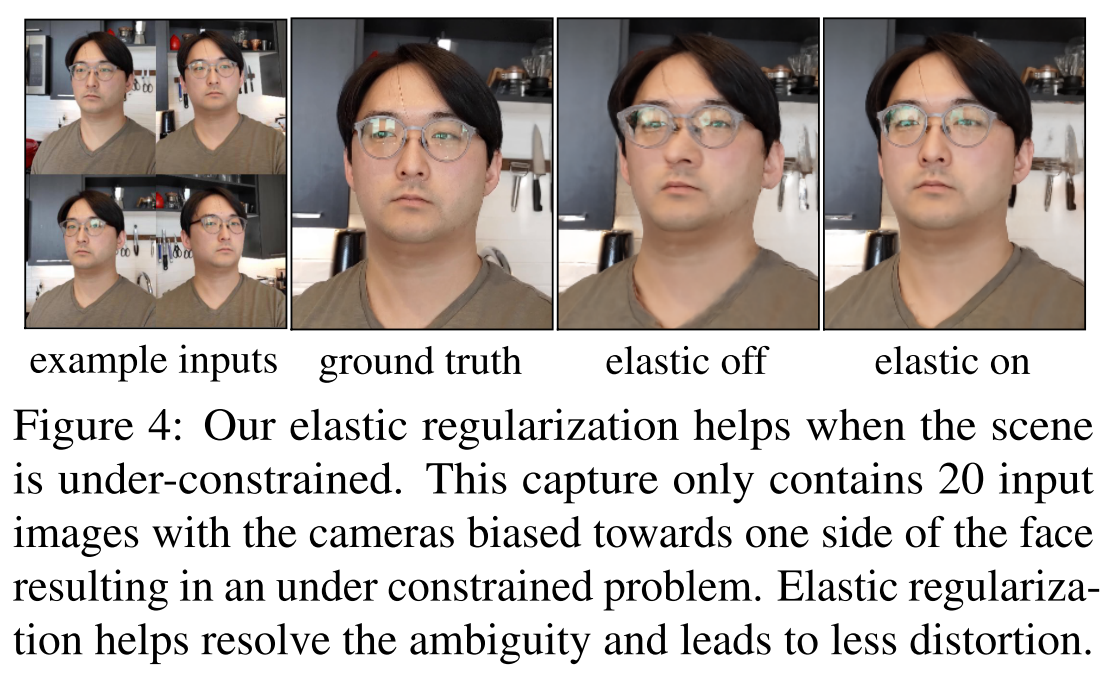# Background Regularization

The deformation field is unconstrained and therefore everything is free to move around. We optionally add a regu- larization term which prevents the background from moving. Given a set of 3D points in the scene which we know should be static, we can penalize any deformations at these points. Given these static 3D points $$\{x_1,\cdots , x_K\}$$, we penalize movement as:

$L_{bg}=\frac{1}{K}\sum_{k=1}^K{\lVert T(x_k)-x_k\rVert_2}$

# Coarse-to-Fine Deformation Regularization

Tancik et al.  show that controls it the smoothness of the network: a low value of m results in a low-frequency bias (low resolution) while a higher value of m results in a higher-frequency bias (high resolution). Consider a motion like in Fig. 5, where subject rotates their head and smiles. With a small m for the deformation field, the model cannot capture the minute motion of the smile; conversely, with a larger m, the model fails to correctly rotate the head because the template overfits to an underoptimized deformation field. To overcome this trade-off, we propose a coarse-to-fine approach that starts with a low-frequency bias and ends with a high-frequency bias.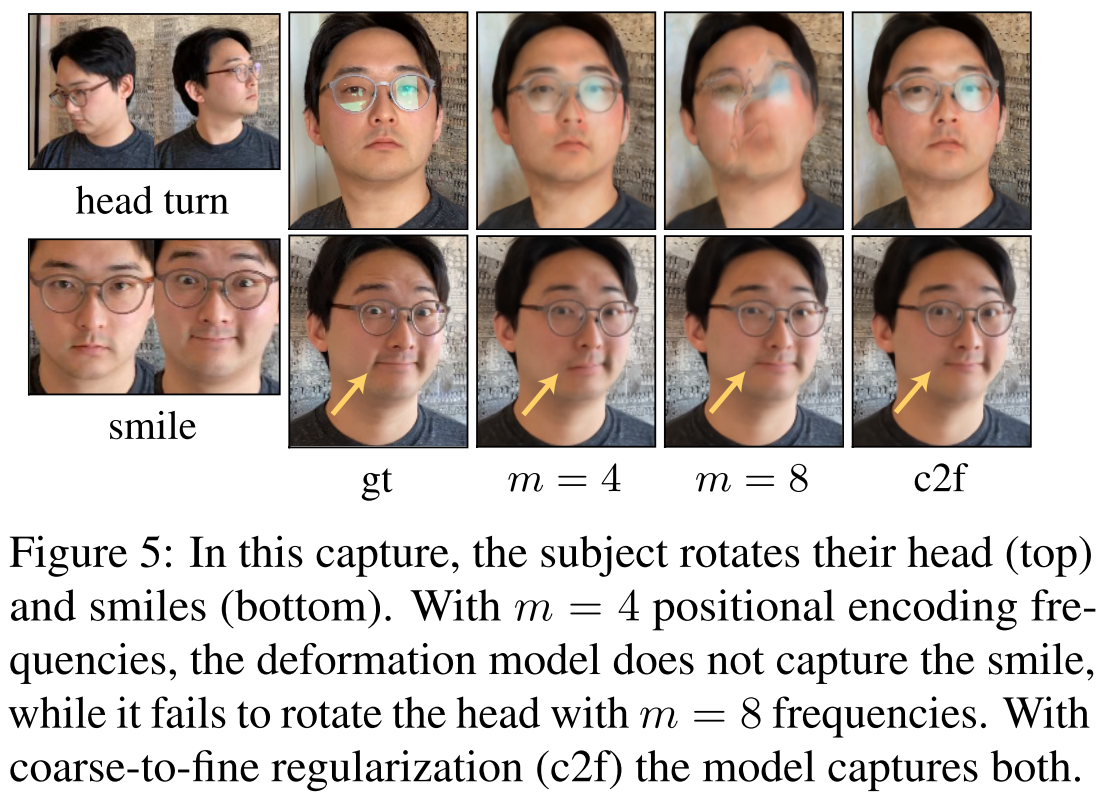As a result, the paper proposes starting with a smaller m to capture the large motion then gradually increases to refine the details. This is achieved by clamping the frequence encoding:

$w_k(\alpha)=\frac{1-\cos(\pi\mbox{ clamp}(\alpha-j,0,1))}{2}\mbox{ where }\alpha(t)=\frac{mT}{N}$

$$\alpha$$ is the clamping factor linearly increased with iteration.

Written on September 26, 2022